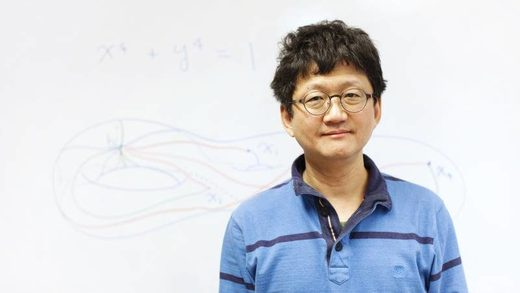© Tom Medwell for Quanta Magazine
Minhyong Kim, a mathematician at the University of Oxford, has long kept his vision to himself. "Number theorists are a pretty tough-minded group of people."
An eminent mathematician reveals that his advances in the study of millennia-old mathematical questions owe to concepts derived from physics.

Mathematics is full of weird number systems that most people have never heard of and would have trouble even conceptualizing. But rational numbers are familiar. They're the counting numbers and the fractions - all the numbers you've known since elementary school. But in mathematics, the simplest things are often the hardest to understand. They're simple like a sheer wall, without crannies or ledges or obvious properties you can grab ahold of.

Minhyong Kim, a mathematician at the University of Oxford, is especially interested in figuring out which rational numbers solve particular kinds of equations. It's a problem that has provoked number theorists for millennia. They've made minimal progress toward solving it. When a question has been studied for that long without resolution, it's fair to conclude that the only way forward is for someone to come up with a dramatically new idea. Which is what Kim has done.

"There are not many techniques, even though we've been working on this for 3,000 years. So whenever anyone comes up with an authentically new way to do things it's a big deal, and Minhyong did that," said Jordan Ellenberg, a mathematician at the University of Wisconsin, Madison.

Over the past decade Kim has described a very new way of looking for patterns in the seemingly patternless world of rational numbers. He's described this method in papers and conference talks and passed it along to students who now carry on the work themselves. Yet he has always held something back. He has a vision that animates his ideas, one based not in the pure world of numbers, but in concepts borrowed from physics. To Kim, rational solutions are somehow like the trajectory of light.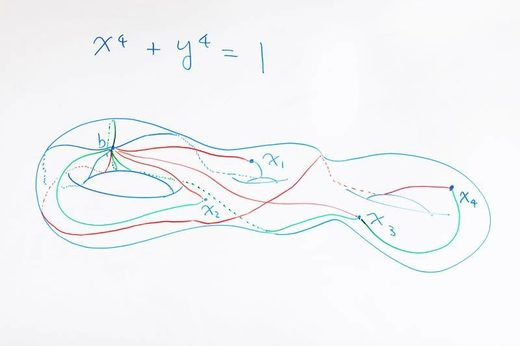© Tom Medwell for Quanta Magazine
A mathematical object called the three-holed torus adorns Kim's whiteboard at the University of Oxford.
If the connection sounds fantastical it's because it is, even to mathematicians. And for that reason, Kim long kept it to himself. "I was hiding it because for many years I was somewhat embarrassed by the physics connection," he said. "Number theorists are a pretty tough-minded group of people, and influences from physics sometimes make them more skeptical of the mathematics."

But now Kim says he's ready to make his vision known. "The change is, I suppose, simply a symptom of growing old!" wrote Kim, 53, in one of the first emails we exchanged for this story.

He has recently hosted a conference that brought together number theorists and string theorists. He has also drafted articles that begin to describe his inspiration to a mathematical community that is not accustomed to thinking about numbers through such direct analogy with the physical world.

Yet one stumbling block remains - a last piece of the physics-math analogy that Kim still has to work out. He hopes that by inviting others into his vision, especially physicists, he'll have the help he needs to complete it.

The Ancient Challenge

Rational solutions to equations exert a strong pull on the human mind. They are satisfying in the way of puzzle pieces falling perfectly into place. For that reason, they are the subject of many of the most famous conjectures in mathematics.

The rational numbers include the integers and any number that can be expressed as a ratio of two integers, such as 1, -4 and 99/100. Mathematicians are particularly interested in rational numbers that solve what are called "Diophantine equations" - polynomial equations with integer coefficients, like x2 + y2 = 1. These equations are named after Diophantus, who studied them in Alexandria in the third century A.D.

Rational solutions are hard to find in any kind of comprehensive way because they don't follow any geometric pattern. Think about that equation x2 + y2 = 1. The real-number solutions to that equation form a circle. Take away all the points on that circle that can't be expressed as a fraction and you're left with all the rational solutions, which don't form such a tidy object. The rational solutions appear to be scattered randomly around the circumference of the circle.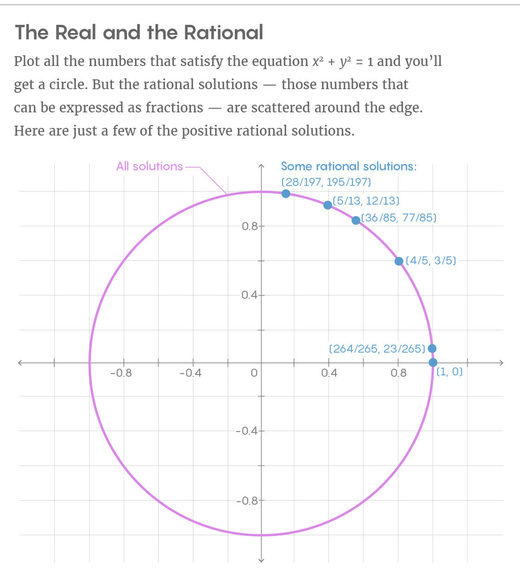"The condition for a point to have rational coordinates is not a geometric condition at all. You can't write an equation that the rational points have to satisfy," Kim said.

It's often easy to find a single rational solution, or even many of them. But mathematicians, who don't like loose ends, are more interested in identifying all the rational solutions. That's much harder. It's so hard, in fact, that proving even the barest statement about the number of rational solutions is enough to make you a mathematical luminary. In 1986 Gerd Faltings won the Fields Medal, math's highest honor, primarily for solving a problem called the Mordell conjecture and proving that certain classes of Diophantine equations have only finitely many rational solutions (rather than infinitely many).© Tom Medwell for Quanta Magazine
Kim in his office at Oxford.
Faltings' proof was a landmark result in number theory. It was also what mathematicians refer to as an "ineffective proof," meaning that it didn't actually count the number of rational solutions, let alone identify them. Ever since, mathematicians have been looking for a way to take those next steps. Rational points look like random points on the ordinary graph of an equation. Mathematicians hope that if they change the setting in which they think about the problem, those points will start to look more like a constellation that they can describe in some precise way. The trouble is, the known land of mathematics doesn't provide such a setting.

"To get effective results on rational points, it definitely has the feeling that there'd have to be a new idea," said Ellenberg.

At present, there are two main proposals for what that new idea could be. One comes from the Japanese mathematician Shinichi Mochizuki, who in 2012 posted hundreds of pages of elaborate, novel mathematics to his faculty webpage at Kyoto University. Five years later, that work remains largely inscrutable. The other new idea comes from Kim, who has tried to think about rational numbers in an expanded numerical setting where hidden patterns between them start to come into view.

A Symmetry Solution

Mathematicians often say that the more symmetric an object is, the easier it is to study. Given that, they'd like to situate the study of Diophantine equations in a setting with more symmetry than the one where the problem naturally occurs. If they could do that, they could harness the newly relevant symmetries to track down the rational points they're looking for.

To see how symmetry helps a mathematician navigate a problem, picture a circle. Maybe your objective is to identify all the points on that circle. Symmetry is a great aid because it creates a map that lets you navigate from points you do know to points you have yet to discover.

Imagine you've found all the rational points on the southern half of the circle. Because the circle has reflectional symmetry, you can flip those points over the equator (changing the signs of all the y coordinates), and suddenly you've got all the points in the northern half too. In fact, a circle has such rich symmetry that knowing the location of even one single point, combined with knowledge of the circle's symmetries, is all you need to find all the points on the circle: Just apply the circle's infinite rotational symmetries to the original point.

Yet if the geometric object you're working with is highly irregular, like a random wandering path, you're going to have to work hard to identify each point individually - there are no symmetry relationships that allow you to map known points to unknown points.

Sets of numbers can have symmetry, too, and the more symmetry a set has, the easier it is to understand - you can apply symmetry relationships to discover unknown values. Numbers that have particular kinds of symmetry relationships form a "group," and mathematicians can use the properties of a group to understand all the numbers it contains.

The set of rational solutions to an equation doesn't have any symmetry and doesn't form a group, which leaves mathematicians with the impossible task of trying to discover the solutions one at a time.

Beginning in the 1940s, mathematicians began to explore ways of situating Diophantine equations in settings with more symmetry. The mathematician Claude Chabauty discovered that inside a larger geometric space he constructed (using an expanded universe of numbers called the p-adic numbers), the rational numbers form their own symmetric subspace. He then took this subspace and combined it with the graph of a Diophantine equation. The points where the two intersect reveal rational solutions to the equation.

In the 1980s the mathematician Robert Coleman refined Chabauty's work. For a couple of decades after that, the Coleman-Chabauty approach was the best tool mathematicians had for finding rational solutions to Diophantine equations. It only works, though, when the graph of the equation is in a particular proportion to the size of the larger space. When the proportion is off, it becomes hard to spot the exact points where the curve of the equation intersects the rational numbers.

"If you have a curve inside an ambient space and there are too many rational points, then the rational points kind of cluster and you have trouble distinguishing which ones are on the curve," said Kiran Kedlaya, a mathematician at the University of California, San Diego.

And that's where Kim came in. To extend Chabauty's work, he wanted to find an even larger space in which to think about Diophantine equations - a space where the rational points are more spread out, allowing him to study intersection points for many more kinds of Diophantine equations.

Tom Medwell for Quanta Magazine

Spaces of Spaces

If you're looking for a larger kind of space, along with clues about how to use symmetry to navigate it, physics is a good place to turn.

Generally speaking, a "space," in the mathematical sense, is any set of points that has geometric or topological structure. One thousand points scattered willy-nilly won't form a space - there's no structure that ties them together. But a sphere, which is just a particularly coherent arrangement of points, is a space. So is a torus, or the two-dimensional plane, or the four-dimensional space-time in which we live.

In addition to these spaces, there exist even more exotic spaces, which you can think of as "spaces of spaces." To take a very simple example, imagine that you have a triangle - that's a space. Now imagine the space of all possible triangles. Each point in this larger space represents a particular triangle, with the coordinates of the point given by the angles of the triangles it represents.

That sort of idea is often useful in physics. In the framework of general relativity, space and time are constantly evolving, and physicists think of each space-time configuration as a point in a space of all space-time configurations. Spaces of spaces also come up in an area of physics called gauge theory, which has to do with fields that physicists layer on top of physical space. These fields describe how forces like electromagnetism and gravity change as you move through space. You can imagine that there's a slightly different configuration of these fields at every point in space - and that all those different configurations together form points in a higher-dimensional "space of all fields."

This space of fields from physics is a close analogue to what Kim is proposing in number theory. To understand why, consider a beam of light. Physicists imagine the light moving through the higher-dimensional space of fields. In this space, light will follow the path that adheres to the "principle of least action" - that is, the path that minimizes the amount of time required to go from A to B. The principle explains why light bends when it moves from one material to another - the bent path is the one that minimizes the time taken.

These larger spaces of spaces that come up in physics feature additional symmetries that are not present in any of the spaces they represent. These symmetries draw attention to specific points, emphasizing, for example, the time-minimizing path. Constructed in another way in another context, these same kinds of symmetries might emphasize other kinds of points - like the points corresponding to rational solutions to equations.

Connecting Symmetry to Physics

Number theory has no particles to track, but it does have something like space-time, and it also offers a way of drawing paths and constructing a space of all possible paths. From this basic correspondence, Kim is working out a scheme in which "the problem of finding the trajectory of light and that of finding rational solutions to Diophantine equations are two facets of the same problem," as he explained last week at a conference on mathematical physics in Heidelberg, Germany.

The solutions to Diophantine equations form spaces - these are the curves defined by the equations. These curves can be one-dimensional, like the circle, or they can be higher-dimensional. For example, if you plot (complex) solutions to the Diophantine equation x4 + y4 = 1, you get the three-holed torus. The rational points on this torus lack geometric structure - that's what makes them hard to find - but they can be made to correspond to points in a higher-dimensional space of spaces that do have structure.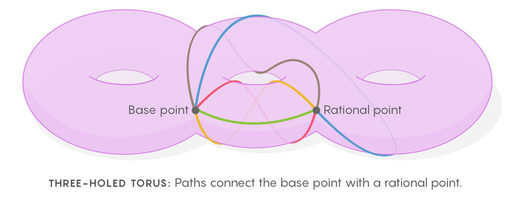Kim creates this higher-dimensional space of spaces by thinking about ways you can draw loops on the torus (or whatever space the equation defines). The loop-drawing procedure goes as follows. First, choose a base point, then draw a loop from that point to any other point and back again. Now repeat that process, drawing paths that connect your base point with every other point on the torus. You'll end up with a thicket of all possible loops that begin and end at the base point. This collection of loops is a centrally important object in mathematics - it's called the fundamental group of a space.

You can use any point on the torus as your base point. Each point will have a unique thicket of paths emanating from it. Each of these collections of paths can then be represented as a point in a higher-dimensional "space of all collections of paths" (like the space of all possible triangles). This space of spaces is geometrically very similar to the "space of spaces" physicists construct in gauge theory: The way collections of paths change as you move from one point to another on the torus strongly resembles the way fields change as you move from one point to another in real space. This space of spaces features additional symmetries not present on the torus itself. And while there is no symmetry between the rational points on the torus, if you go into the space of all collections of paths, you can find symmetries between the points associated to the rational points. You gain symmetries that were not visible before.

"A phrase I use sometimes is that there is a kind of 'hidden arithmetic symmetry' encoded in these paths that is highly analogous to the internal symmetries of gauge theory," Kim said.

Just as Chabauty did, Kim finds rational solutions by thinking about intersection points in this larger space he's constructed. He uses symmetries of this space to narrow in on the intersection points. His hope is to develop an equation that detects these points exactly.

In the physics setting, you can imagine all possible paths that a ray of light could take. This is your "space of all paths." The points in that space that interest physicists are the points corresponding to time-minimizing paths. Kim believes the points corresponding to thickets of paths emanating from rational points have something of this same quality - that is, the points minimize some property that comes up when you start to think about the geometric form of Diophantine equations. Only he hasn't yet figured out what that property might be.

"What I started out trying to find" was a least-action principle for the mathematical setting, he wrote in an email. "I still don't quite have it. But I am pretty confident it's there."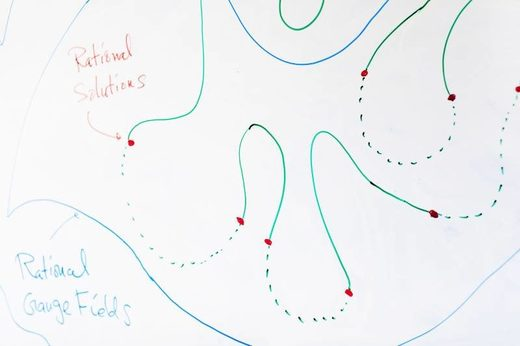© Tom Medwell for Quanta Magazine
An Uncertain Future

Over the past few months I've described Kim's physics-inspired vision to several mathematicians, all admirers of Kim's contributions to number theory. When presented with this take on his work, however, they didn't know what to make of it.

"As a representative number theorist, if you showed me all the awesome stuff Minhyong has been doing and asked me if this was physically inspired, I'd say, 'What the hell are you talking about?'" Ellenberg said.

So far, Kim has made no mention of physics in his papers. Instead, he's written about objects called Selmer varieties, and he's considered relationships between Selmer varieties in the space of all Selmer varieties. These are recognizable terms to number theorists. But to Kim, they've always been another name for certain kinds of objects in physics.

"It should be possible to use ideas from physicists to solve problems in number theory, but we haven't thought carefully enough about how to set up such a framework," Kim said. "We're at a point where our understanding of physics is mature enough, and there are enough number theorists interested in it, to make a push."

The primary obstacle to the development of Kim's method lies in the search for some kind of action to minimize in the space of all thickets of loops. This kind of perspective comes naturally in the physical world, but it doesn't make any obvious sense in arithmetic. Even mathematicians who follow Kim's work closely wonder whether he'll find it.

"I think [Kim's program] is going to do a lot of great things for us. I don't think we're going to get as sharp an understanding as Minhyong wants where rational points are honestly classical solutions to some kind of arithmetic gauge theory," said Arnav Tripathy, a professor of mathematical physics at Harvard University.

Today the language of physics remains almost entirely outside the practice of number theory. Kim thinks that's almost certainly going to change. Forty years ago, physics and the study of geometry and topology had little to do with one another. Then, in the 1980s, a handful of mathematicians and physicists, all towering figures now, found exact ways to use physics to study the properties of shapes. The field has never looked back.

"It's almost impossible to be interested in geometry and topology nowadays without knowing something about [physics]. I'm reasonably sure this will happen with number theory" in the next 15 years, Kim said. "The connections are so natural."